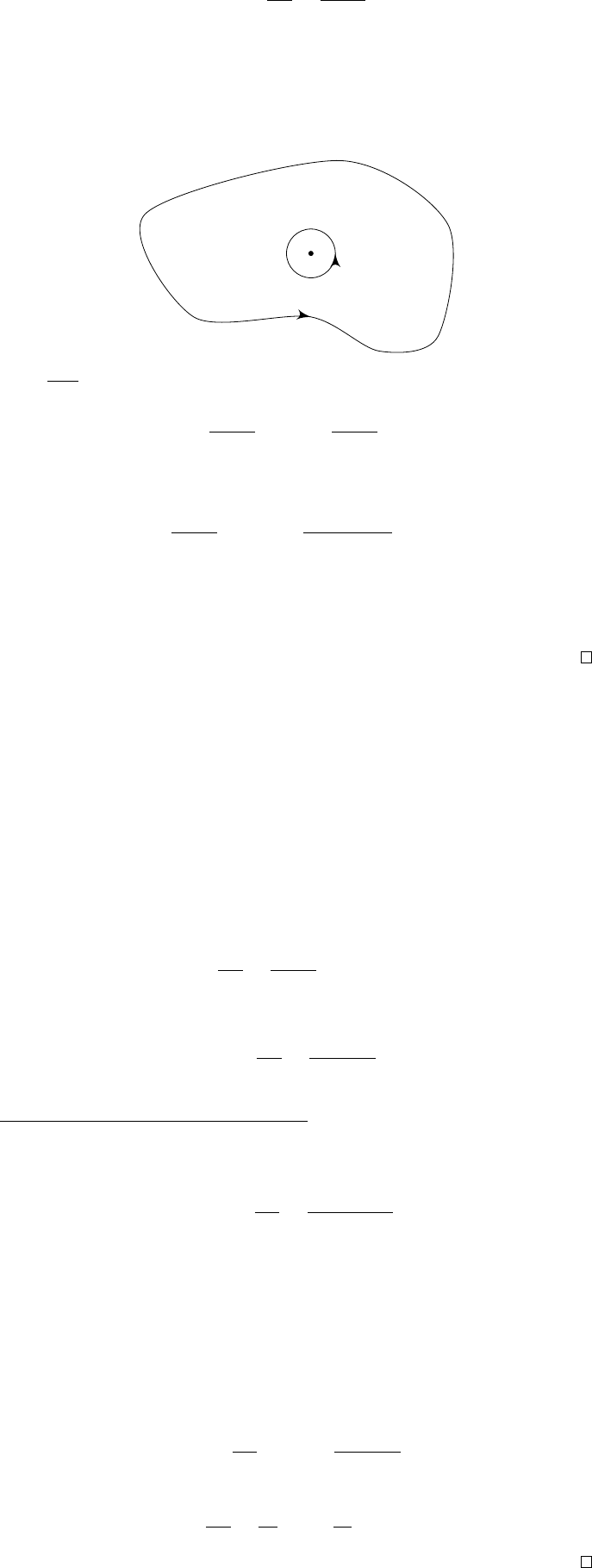2Contour integration and Cauchy's theorem

IB Complex Methods2.4 Cauchy’s integral formula
Theorem
(Cauchy’s integral formula)
.
Suppose that
f
(
z
) is analytic in a domain
D and that z
0
D. Then
f(z
0
) =
1
2πi
I
γ
f(z)
z z
0
dz
for any simple closed contour γ in D encircling z
0
anticlockwise.
This result is going to be very important in a brief moment, for proving one
thing. Afterwards, it will be mostly useless.
Proof. (non-examinable) We let γ
ε
0
, within γ.
z
0
γ
ε
γ
Since
f(z)
zz
0
is analytic except when z = z
0
, we know
I
γ
f(z)
z z
0
dz =
I
γ
ε
f(z)
z z
0
dz.
We now evaluate the right integral directly. Substituting z = z
0
+ εe
, we get
I
γ
ε
f(z)
z z
0
dz =
Z
2π
0
f(z
0
+ εe
)
εe
iεe
dθ
= i
Z
2
0
π(f(z
0
) + O(ε)) dθ
2πif(z
0
)
as we take the limit ε 0. The result then follows.
So, if we know
f
on
γ
, then we know it at all points within
γ
. While this
seems magical, it is less surprising if we look at it in another way. We can write
f
=
u
+
iv
, where
u
and
v
are harmonic functions, i.e. they satisfy Laplace’s
equation. Then if we know the values of
u
and
v
on
γ
, then what we essentially
have is Laplace’s equation with Dirichlet boundary conditions! Then the fact
that this tells us everything about
f
within the boundary is just the statement
that Laplace’s equation with Dirichlet boundary conditions has a unique solution!
The difference between this and what we’ve got in IA Vector Calculus is that
Cauchy’s integral formula gives an explicit formula for the value of
f
(
z
0
), while
in IA Vector Calculus, we just know there is one solution, whatever that might
be.
Note that this does not hold if
z
0
does not lie on or inside
γ
, since Cauchy’s
theorem just gives
1
2πi
I
γ
f(z)
z z
0
dz = 0.
Now, we can differentiate Cauchy’s integral formula with respect to
z
0
, and
obtain
f
0
(z
0
) =
1
2πi
I
γ
f(z)
(z z
0
)
2
dz.
We have just taken the differentiation inside the integral sign. This is valid since
it’s Complex Methods and we don’t care
the integrand, both before and after,
is a continuous function of both z and z
0
.
We see that the integrand is still differentiable. So we can differentiate it
again, and obtain
f
(n)
(z
0
) =
n!
2πi
I
γ
f(z)
(z z
0
)
n+1
dz.
Hence at any point
z
0
where
f
is analytic, all its derivatives exist, and we have
just found a formula for them. So it is differentiable infinitely many times as
A classic example of Cauchy’s integral formula is Liouville’s theorem.
Theorem (Liouville’s theorem*). Any bounded entire function is a constant.
Proof.
(non-examinable) Suppose that
|f
(
z
)
| M
for all
z
, and consider a circle
of radius r centered at an arbitrary point z
0
C. Then
f
0
(z
0
) =
1
2πi
I
|zz
0
|=r
f(z)
(z z
0
)
2
dz.
Hence we know
1
2πi
1
2π
· 2πr ·
M
r
2
0
as r . So f
0
(z
0
) = 0 for all z
0
C. So f is constant.